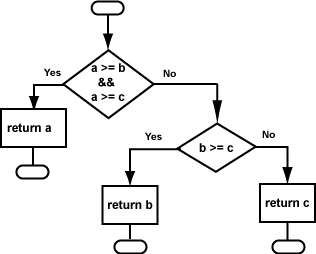If the maximum is `a`, where in the code is this known?

The first statement after the first IF .

# Another VersionIt seems a little silly that the maximum `a` is not returned until the last line of the function when it is known so early. In this situation, most C programmers would return `a` as soon as it is known to be the maximum. The flowchart at right shows this.

Here is the code that corresponds to this flowchart:

```/*
pre-condition: a, b, and c are integers.
exit condition: return the maximum of a, b, and c
*/
int maxThree(int a, int b, int c)
{
if (a >= b && a >= c) return a;
if (b >= c) return b;
return c;
}
```

It is likely that you find both the new flowchart and the new function straightforward and easier to understand than the previous versions.

### QUESTION 3:

Is the new flowchart (and the new function) structured?